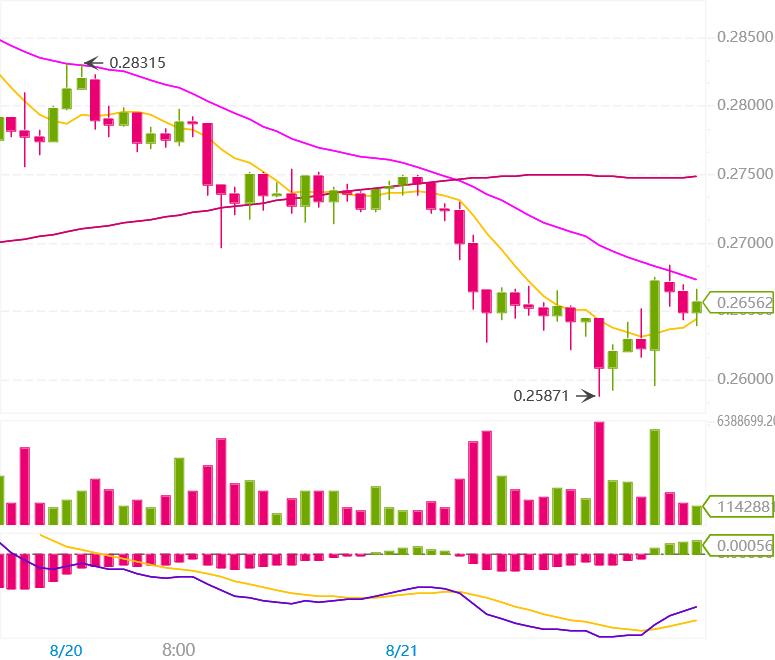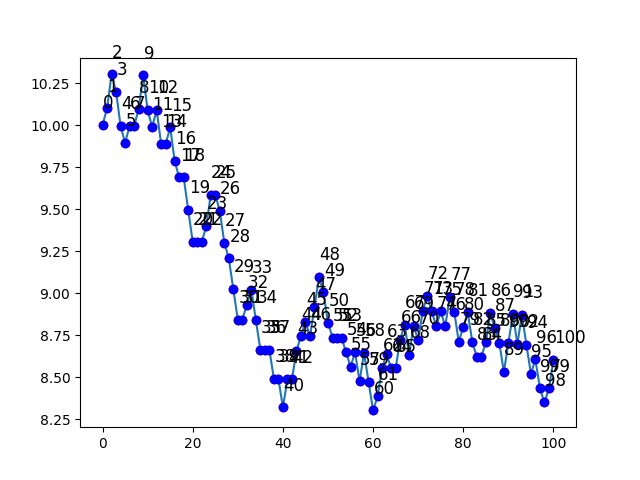# whydoitweet

## Small Sims (2): Simulating a crypto price chartLast time in Small Sims we looked at simulating people walking in random directions. Today we are going to take one step further, and build on the things we put together in that last post to make a very basic simulation of the price movement of a cryptocurrency. Think of generating an chart a bit like this:

(image courtesy of Binance.com)Like last time, we'll use repl.it to make this simulation.

So our mission is:

• to make a simple simulation that can make a jittery curve like this, reproducing a typical crypto price curve.

In this post I will explain you a way how to make this, and you can follow it in two ways:

1. If you want to follow it as an actual tutorial, then create a new repl, and add code to your main.py file whenever you see blocks like:

`#This is an example comment.`

2. Or, you can simply read/skim/browse the post, and download a working repl.it example at the end, which you can then test and modify as you see fit :).

## What libraries do we need today?

To make our lives much easier, we will need to paste in all the code from the previous Random Walk post into our main.py file.

We do need to comment out the last line though, to prevent the original Random Walk simulation from being run.

## From a Random Walk to a Random Price Change algorithm

Remember, the Random Walk worked like this:

• As a starting point you have an object (e.g., an NPC) on a given location.

Then, at every time step the object:

- picks a random direction (North, South, East or West)

- and moves one step in that direction.

Now to move the price, we need to reinterpret the x and y coordinates. In the graph above, the time is on the x axis, while the price is on the y axis. To start of, we make a point at x=0, with a starting price of say, y=\$10.

Every step of the simulation, we want to increment the time by one (so x +=1), and we want to change the price by, say, up to 2% (so somewhere between y *= 0.98 and y *= 1.02). To do this, we need to change the “directions” in our Random Walk algorithm, such that instead of going North, East, South or West, we go +2%,+1%,unchanged,-1% or -2%. In code, such a changed step function looks like this:

`def random_price_change(xs, ys):`

`direction = random.randint(-2, 2)`

`newx = xs[-1] + 1`

`# time x is incremented by 1`

`newy = (ys[-1] * (100.0 + direction)) / 100.0`

`# y is multiplied by a value in the range of 0.98 to 1.02.`

`xs.append(newx)`

`ys.append(newy)`

## Making a random price progression, using the algorithm

Just like last time, making the chart is just a matter of calling the same function many times. You can do this as follows:

`def random_price_chart_simple(x_start, y_start, steps=10):`

`xsprice = [x_start]`

`ysprice = [y_start]`

`for i in range(0,steps):`

`random_price_change(xsprice, ysprice)`

`return xsprice,ysprice`

Of course, making the function alone will not give you any output when you run the script. To actually use the function and generate a price graph with 100 time points, you can use:

`xsprice,ysprice = random_price_chart_simple(0,10, steps=100)`

And to print out the values in text, just use:

`print(xsprice,ysprice)`

## Plotting the output of your simulation

The main reason why it's useful to reuse the code from my previous post, is because the plotting is very similar. You can actually re-use the plot_random_walk() function without any further modifications for this. Just add:

`plot_random_walk(xsprice,ysprice,name='random_price_chart.png')`

(I changed the filename of the output to something more appropriate for this post)

Now remember: due to a glitch in repl.it, you should delete your output random_price_chart.png file every time you rerun your code. If not, you may risk generating a corrupted image.

If you run the code, you will obviously get a random graph. Mine looks like this:Which by coincidence is not too far off from the chart above :).

By the way, if the numbers near the point annoy you, you could remove them by commenting out these lines in plot_random_walk().

`for i in range(len(xswalk)):`

`x = xswalk[i]`

`y = yswalk[i]`

`plt.plot(x, y, 'bo')`

`plt.text(x + 0.1, y + 0.1 , i, fontsize=12)`

## Closing thoughts

The main point of this post is to show how you can make a very basic change to an existing algorithm to use it for something rather difference. In addition, I chose this topic because I am curious whether people are interested in simulating price curves. I suppose I can gauge the interest by the upvotes this post gets in comparison to others, and then take that into account when choosing topics for future Small Sim editions :).

Just like last time, I will leave you with:

1. two questions for the experts.

2. an overview of the full code (and a link to the repl I made).

3. (only for Coil subscribers): some new suggestions on improving Coil and Coil.com.

Previous: Random Walk.

Next: Living Populations.

## Appendix 1: Expert question

Here's two questions to challenge the experts:

• Can you make the random graph generator such that it generates exactly the same graph for everyone who is using your program?
• How would you incorporate “whales” in your simulation, who could periodically pump the price up by a large amount, and then dump at an arbitrary later moment?

## Appendix 2: Code summary

So in conclusion, the full code will look like this (note that you could choose to leave the random_step and the random_walk functions out from the code if you prefer):

`### BEGIN OF PREVIOUS RANDOM WALK EXAMPLE`

`# we need to generate random numbers`

`import random`

`# and we need to plot stuff.`

`import matplotlib.pyplot as plt`

`import matplotlib as mpl`

`mpl.use('Agg')`

`# define a function that takes one step in a random direction`

`# we assume 4 possible directions: N,E,S,W.`

`def random_step(xs, ys):`

`direction = random.randint(0,3)`

`newx = xs[-1]`

`newy = ys[-1]`

`if direction == 0:`

`newx -= 1`

`if direction == 1:`

`newx += 1`

`if direction == 2:`

`newy -= 1`

`if direction == 3:`

`newy += 1`

`xs.append(newx)`

`ys.append(newy)`

`def random_walk(x_start, y_start, steps=10):`

`xswalk = [x_start]`

`yswalk = [y_start]`

`for i in range(0,steps):`

`random_step(xswalk, yswalk)`

`return xswalk,yswalk`

`# Let's try it out!`

`xswalk,yswalk = random_walk(100,100)`

`print(xswalk,yswalk)`

`def plot_random_walk(xswalk, yswalk, name='random_walk.png'):`

`plt.clf()`

`#plot the lines`

`plt.plot(xswalk, yswalk)`

`#plot the points`

`plt.plot(xswalk, yswalk, 'ro')`

`# add a step number near each point.`

`for i in range(len(xswalk)):`

`x = xswalk[i]`

`y = yswalk[i]`

`plt.plot(x, y, 'bo')`

`plt.text(x + 0.1, y + 0.1 , i, fontsize=12)`

`plt.show()`

`plt.savefig(name)`

`# Let's try to plot our random walk`

`# plot_random_walk(xswalk,yswalk) ## commented out because we don't want to run the random walk example here.`

`### END OF PREVIOUS RANDOM WALK EXAMPLE`

`# Now we make a variation for a price chart.`

`def random_price_change(xs, ys):`

`direction = random.randint(-2, 2)`

`newx = xs[-1]+1`

`newy = (ys[-1] * (100.0 + direction)) / 100.0`

`xs.append(newx)`

`ys.append(newy)`

`def random_price_chart_simple(x_start, y_start, steps=10):`

`xsprice = [x_start]`

`ysprice = [y_start]`

`for i in range(0,steps):`

`random_price_change(xsprice, ysprice)`

`return xsprice,ysprice`

`# Let's try it out!`

`xsprice,ysprice = random_price_chart_simple(0,10, steps=100)`

`print(xsprice,ysprice)`

`plot_random_walk(xsprice,ysprice,name='random_price_chart.png')`

And a working repl.it of it can be found here.

Continue reading with a Coil membership.